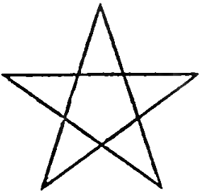# Homework 3: Circle Logic

### Short Description:

Create programs which employs the ideas from the circle notes.

### Goals

When you finish this homework, you should
• Understand how to generate a regular polygon
• Understand how a regular polygon can be used to approximate a circle.

### Formal Description

1. Create a program and interface that allows a user to explore the accuracy of a circle drawn as a regular n-gon.

Your program should have a canvas which displays a "circle" like object centered in the middle, with diameter 90% of the minimum dimension of the canvas. To test this provide the user with the ability to reset the canvas size at runtime.

You should draw a "circle" based on an input provided by the user. This should be a single integer, the number of sides to use in the regular polygon to approximate the circle. This number should be a minimum of three and may be as large as you wish within the following constraint. You should never attempt to draw a line segment with length less than 1 pixel.

For example, if the user enters a 3, the screen should show a circle with an equilateral triangle where each vertex of the triangle is on the circle.

Expected output

• A circle, drawn with ctx.arc() with the given diameter centered on the middle of the canvas.
• A regular polygon inscribed in the above circle. The sides of this polygon should be determined by the user input.
• The circumference of the circle.
• The perimeter of the polygon.
• The ratio of these two, use the circle for the denominator.
• The average length of each polygon segment.
2. Create a program that will draw an approximation of a spiral.

This should be fairly simple. I would start at the center of the screen, with $\Theta = 0, r = 0$. Loop until the point is outside of the screen changing the $\Theta$ by a fixed amount (probably provided by the user) and $r$ by a fixed amount (again provided by the user).

If you want, you might change $r$ some way other than linearly. Make something that looks nice. (And not too expensive.)

3. Challenge (Optional, but I will give extra points), Create a five sided star algorithmically. This star should be centered on the canvas with "radius" of 90% of the canvas.4. Double challenge (Double optional, but again I will give extra points): Make this star rotate and twinkel (grow and shrink slightly) with a timer.

Note, this should be done as parameter(s) to computing the star, not with a rotation matrix. Think offset in size and the angle $\Theta$.

### Documentation

Please use relative references to your code in the html file. This will allow me to extract the file in my local environment.